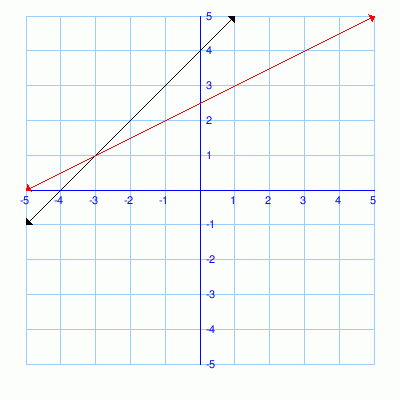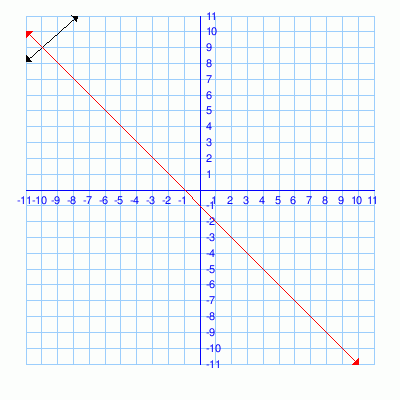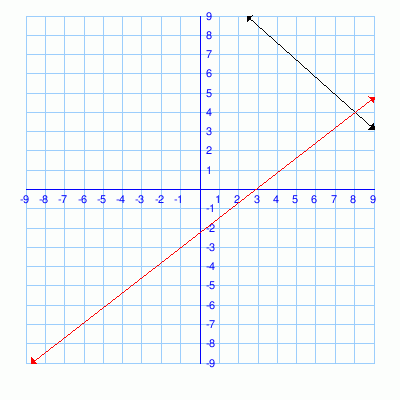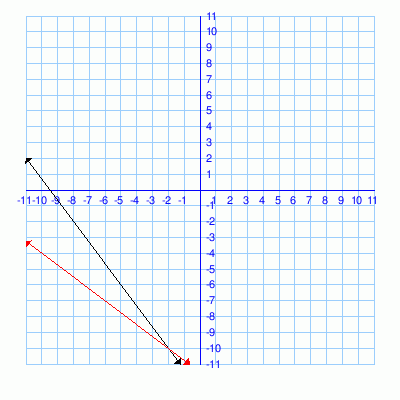# Math Practice Topic: System of Linear Graphs

 Description: Solve a system of linear equations from the graphs. Adaptive Learning Progression: The scale of the graphs gets bigger. Start using MathScore for free

## Sample Levels (out of 6)

Solve using the graph. Answer in the form (x,y) -- using parentheses. For example: (-2,3)

1.

y = x + 4
 y
=
 x2
 +
 52Solve using the graph. Answer in the form (x,y) -- using parentheses. For example: (-2,3)

1.

 y
=
 7x8
 +
 714

y = -x + - 12.

 y
=
 -9x10
 +
 565

 y
=
 7x9
 +
 -2093.

 y
=
 -4x3
 +
 -383

 y
=
 -3x4
 +
 -232